# Humming Bird - HMO PDF Sample Papers for Class 1

Resources:

Class 1 sample paper & practice questions for Humming Bird Mathematics Olympiad (HMO) level 1 are given below. Syllabus for level 1 is also mentioned for these exams. You can refer these sample paper & quiz for preparing for the exam.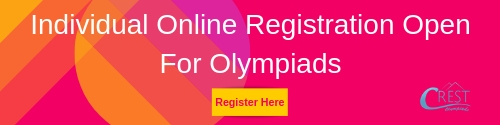Sample Questions from Olympiad Success:

 Q.1 Q.2 Q.3 Q.4 Q.5 Q.6 Q.7 Q.8 Q.9 Q.10
 Q.1 Multiply the given numbers: 7 x 9 = ………………. a) 80 b) 50 c) 63 d) 70
 Q.2 What is the sum of all sides given in the figure?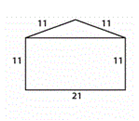a) 44 b) 55 c) 65 d) 75
 Q.3 Arrange the numbers from lowest to highest. 12, 56, 75, 36, 4 a) 12,4,36,75,56 b) 4,12,36,56,75 c) 4,12,56,36,75 d) 75,56,36,12,4
 Q.4 The number "0" is _____ in shape. a) Oval b) Square c) Rectangle d) Triangle
 Q.5 Write 64 in words. a) Sixty four b) Fifty five c) Seventy five d) Forty five
 Q.6 What is the name of given shape?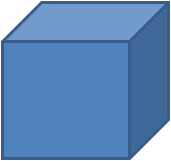a) Square b) Rectangle c) Cuboid d) Cube
 Q.7 Solve the equation: 20 + 19 - 59 + 30 = …………… a) 15 b) 10 c) 98 d) 12
 Q.8 How many books are there in the given figure?a) 12 b) 7 c) 10 d) 16
 Q.9 What is the time?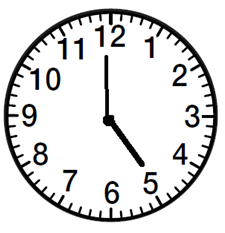a) 4:00 b) 3:10 c) 5:00 d) 5:55
 Q.10 Count the circles, write the numbers and find the sum.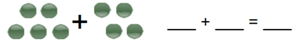a) 4 + 5 = 9 b) 4 + 4 = 8 c) 5 + 4 = 9 d) 5 + 4 = 10Sample PDF of Humming Bird - Humming Bird Mathematics Olympiad (HMO) PDF Sample Papers for Class 1: